Courses
Courses for Kids
Free study material
Free LIVE classes
More

# Turning ForcesLIVE
Join Vedantu’s FREE Mastercalss

## Overview of Turning Forces

To turn or spin something around, forces are required. Moving objects move in straight directions when left unattended. Instead, turning them in a circle requires a force. Larger forces are required to rotate larger objects. Make them spin faster or in smaller, more precise circles.

Real-world examples of the turning effect of forces include pushing a door open, swinging on a see-saw, and pedalling a bicycle. When you can understand what actually occurs in real life, it appears to be rather straightforward. We will learn more about turning forces in this article. Let’s dive in and learn about turning forces.Two Children on a see-saw

## Relate Problems to Real-life Scenarios

There are a few highly prevalent instances of the forces turning in our daily lives. It will be simpler to visualise what is happening if you try to draw comparisons between the questions on your worksheet and real-world situations we have encountered. If you're still not sure what the turning effect of forces is all about, think of a see-saw.

You are aware that two equal-weight individuals must sit at either end of the see-saw to keep it balanced. You could claim that both forces are exerting the same amount of pressure on the see-saw.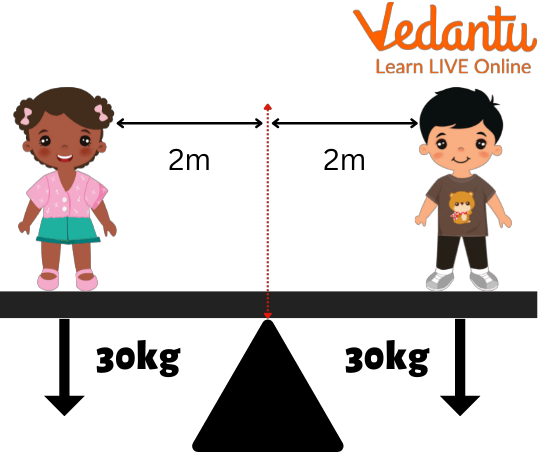Turning Effect on a see-saw

But what if one of the people—with the same weight!—was moved closer to the see-pivot saw's point? The person's side will incline downward, appearing heavier. Take note of the fact that the distance from the pivot, in addition to weight, affects how much a difference there is.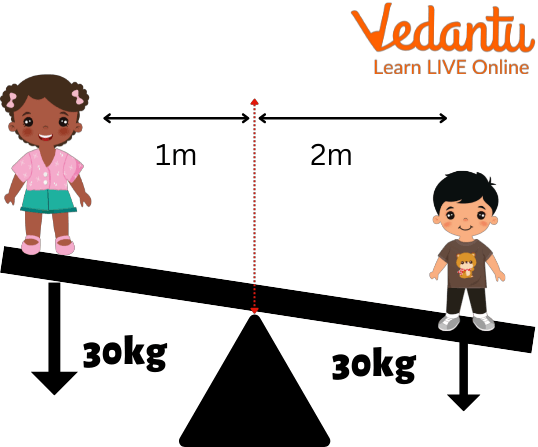One Kid Moved Closer to the Centre

Consider how you could balance the see-saw while maintaining the same positions as in the second scenario. One method is to put more weight on the person closest to the pivot.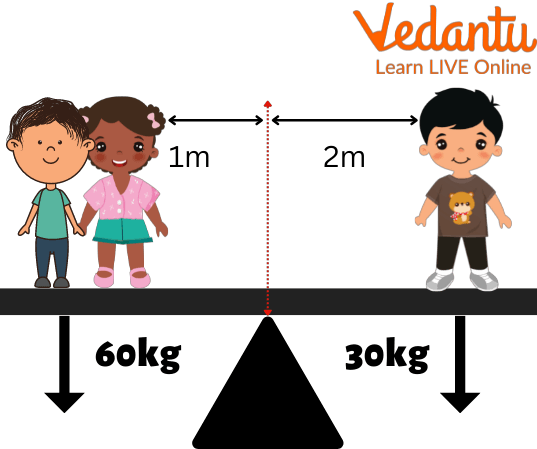More Weight on One Side

## The Formula for Turning Effect of Forces

As you can see, two factors—distance from the pivot and weight of the person—influence the force acting upon the see-saw.

Observation 1: The turning effect rises with distance from the pivot for an equal weight applied.

Observation 2: As the weight applied rises, the turning effect grows at a constant distance from the pivot.

Putting two and two together, we arrive to the following equation:

Weight (N) x Distance (m) = Turning effect (Nm)

It's crucial to understand that the turning effect of forces is also known as moments and, occasionally, torque.

torque = moment of force x turning effect

## Effects of Force

An object can change its size or shape, begin moving, stop moving, accelerate, or decelerate as a result of a force acting on it. The combined effect of all the forces acting on an object is known as the "resultant force," and it is the same as if only one force were to be operating on the object in that direction. To alter an object's velocity, the resulting force is necessary.

• The forces acting on the item are balanced if the resultant force is zero.

• The object will remain stationary if the resultant force exerted on it is "zero." In this scenario, the object will go straight ahead at a constant pace.

• The object will either accelerate or decelerate if the net force acting on it is not zero.

Force is the push or pull that an item with that mass feels and that results in the change in velocity. Newton is the SI unit of force.

F = m x a

Mass is m and acceleration is a.

A body at rest can be forced to move. It can either slow down or stop a moving body. It can quicken the pace of a moving object, and can alter the direction of a moving body as well.

## Moment of a Force

An object may pivot about a point due to a force.

The moment of the force is the term used to describe a force's turning effect.

Moments can move clockwise or counterclockwise around a pivot.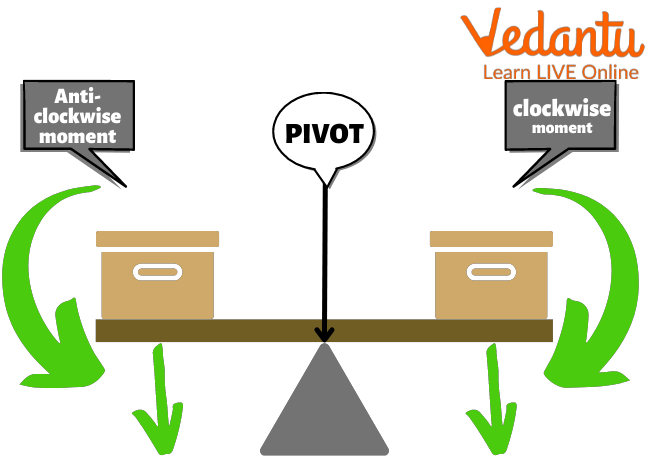Moment of a Force

The anticlockwise moment acts downward on the left, and the clockwise moment acts downwards on the right.

## Calculating the Moment of a Force

The size of the moment of a force can be calculated using the equation:

moment of a force = force (F) x perpendicular distance from the pivot (d)

moment = F x d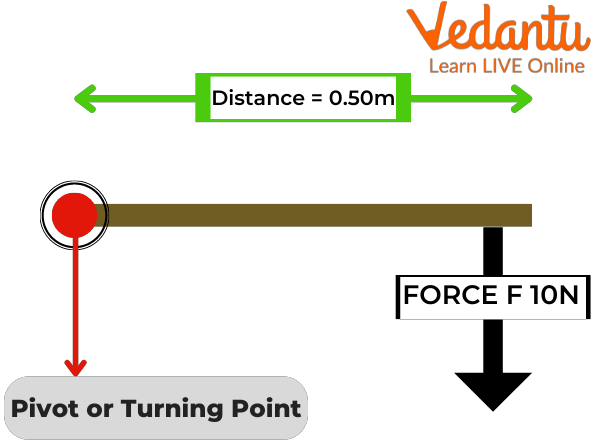Calculation of Moment of Force

Perpendicular distance from pivot to force d = 0.50 m.

Force F = 10 N

Moment = Fd

Moment = 10 N x 0.50 m

Moment = 5 Nm

This is a clockwise moment.

## What is Turning Effect of a Force?

The term "moment of force" or "torque" refers to the action of a force on a rigid body that causes it to rotate around a point, or fulcrum. It is calculated by multiplying the force by the pivot's perpendicular distance from the direction of the force. Newton-metre is its SI equivalent (Nm).

## Factors Affecting Turning Effect of Forces

The turning effects of the force depend on a number of variables. These elements are listed below:

• The magnitude of applied force.

• The applied force and the moment of force are directly proportional.

• The perpendicular distance from the pivoted end.

The moment of force is significantly influenced by the angle of perpendicularity between the applied force and the pivot point. It is challenging to generate a big moment of force if the distance between the applied force and the pivot point is less.

For example: A door handle is subjected to a 15 N force at a distance of 12 cm from the hinge. Calculate the moment of force or turning effect of force

Sol: Perpendicular distance from pivot to force d = 12 cm = 0.12 m.

Turning force F = 15 N.

Moment = Fd.

Moment = 15 N x 0.12 m.

Moment = 1.8 Nm.

## Summary

This article has shown us that using force in daily life is fairly common. In order to move a given body at a specific speed or direction, lift objects, throw a cricket ball, or walk on a road, we employ force. The many consequences of force are all quite well known to us. We are able to push and pull. Once you understand the underlying concepts, the turning effect of force is not a difficult subject to study! The remaining steps essentially consist of reading the question attentively and making the relevant calculations. We hope you enjoyed reading this article, in case of any other doubts, feel free to ask in the comments.

Last updated date: 25th Sep 2023
Total views: 96.6k
Views today: 2.96k

## FAQs on Turning Forces

1. What are the five types of forces?

Gravitational force, electric force, frictional force, nuclear force and magnetic are the five types of forces.

2. Give one example of frictional force acting?

Walking on the road is one example of frictional force. It happens in a way that while walking friction holds our shoe on the ground, and helps us in walking. There is a reason why we tend to slip on well-polished grounds, because they have less friction.

3. What does magnetic force create?

Magnetic force creates magnetic field.